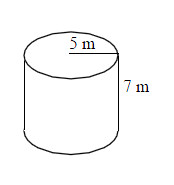### Home > MC1 > Chapter 9 > Lesson 9.3.4 > Problem9-158

9-158.

Calculate the volume of the cylinder pictured at right. Round your answer to the nearest tenth.

The volume of a cylinder is equal to $\left(πr^{2}\right)h$.

$V = \left(π5^{2}\right)\left(7\right) = \left(25\right)\left(7\right)π = 175π$, which is about $549.5 \text {m}^{3}$.